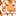# New to Qlik Sense

If you’re new to Qlik Sense, start with this Discussion Board and get up-to-speed quickly.

Announcements
LINKEDIN LIVE: Democratizing data to enhance customer-centricity. JULY 29TH REGISTER TODAY
cancel
Showing results for
Did you mean:Contributor

## Issue with Accumulation in Qlik Sense

Hi Team,

I am working on Line chart and i wanted to show accumulated values between months. But the calculation of the measure includes many measures so it is not working as expected with RangeSum().

Working fine with the below expression (example 1):

=RangeSum(above(sum({<[%DATE]={">=\$(vYearStartDate)<=\$(vYearEndDate)"}>}CURR_EFF1_1),0,RowNo()))

Not working perfectly as expected with the below example as it includes many measures in it (Example :2):

=RangeSum(Above((Sum({<[%DATE]={">=\$(vYearStartDate)<=\$(vPM)"}>}SPEND_EUR_K)-
Sum({<[%DATE]={">=\$(vYearStartDate)<=\$(vPM)"}>} CURR_EFF1_1)-
Sum({<[%DATE]={">=\$(vYearStartDate)<=\$(vPM)"}>} CURR_EFF1_2)-
Sum({<[%DATE]={">=\$(vYearStartDate)<=\$(vPM)"}>} CKF_MVMPOT002_QTY_EFF_1)-
Sum({<[%DATE]={">=\$(vYearStartDate)<=\$(vPM)"}>} QTY_SAV_EUR)-
Sum({<[%DATE]={">=\$(vYearStartDate)<=\$(vPM)"}>} RKF_MVMPOT002_PORT_EFF_1)-Sum(NET_PROJ_COST)-
Sum({<[%DATE]={">=\$(vYearStartDate)<=\$(vPM)"}>}SPEND_EUR_K)-
(Sum({<[%DATE]={">=\$(vYearStartDate)<=\$(vPM)"}>}PRI_SAV_KPI_REL_EUR)-
Sum({<[%DATE]={">=\$(vYearStartDate)<=\$(vPM)"}>}CURR_EFF1_PS))
),0,RowNo()))

Please check the Example 2 and help me with the correct way.

cheers,

Nagesh M.

Labels (2)

• ### SaaS